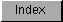```
afp()    FLOAT afp( BYTE *string );
Convert ASCII string into FFP equivalent.

arnd()   VOID arnd( LONG place, LONG exp, BYTE *string);
Round ASCII representation of FFP number.

dbf()    FLOAT dbf( ULONG exp, ULONG mant);
Convert FFP dual-binary number to FFP equivalent.

fpa()    LONG fpa( FLOAT fnum, BYTE *string);
Convert FFP variable into ASCII equivalent.

Be sure to include proper data type definitions, as shown in the example
below.  Print statements have been included to help clarify the format of
the math conversion function calls.

mathffp.c

```